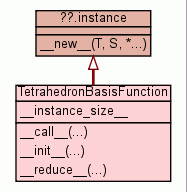[frames] | no frames]

# Class TetrahedronBasisFunctionInstance Methods

 __call__(...) __call__( (TetrahedronBasisFunction)arg1, (numpy.ndarray)arg2) -> float :

 __init__(...) __init__( (object)arg1, (object)arg2, (object)arg3, (object)arg4) -> None :

 __reduce__(...) helper for pickle

Inherited from `unreachable.instance`: `__new__`

Inherited from `object`: `__delattr__`, `__getattribute__`, `__hash__`, `__reduce_ex__`, `__repr__`, `__setattr__`, `__str__`

 Class Variables
__instance_size__ = `200`
 Properties

Inherited from `object`: `__class__`

 Method Details

### __call__(...)(Call operator)

```
__call__( (TetrahedronBasisFunction)arg1, (numpy.ndarray)arg2) -> float :

C++ signature :
double __call__(hedge::tetrahedron_basis_function {lvalue},pyublas::numpy_vector<double>)

```

### __init__(...)(Constructor)

```
__init__( (object)arg1, (object)arg2, (object)arg3, (object)arg4) -> None :

C++ signature :
void __init__(_object*,unsigned int,unsigned int,unsigned int)

```
Overrides: object.__init__

### __reduce__(...)

helper for pickle

Overrides: object.__reduce__
(inherited documentation)

 Generated by Epydoc 3.0.1 on Sat Aug 29 14:33:14 2009 http://epydoc.sourceforge.net